Voltage doubler uses inherent features of push-pull dc/dc converter

Allegro ULN2023A

Ajoy Raman

EDN

This Design Idea presents a minimal-parts-count, wide-range voltage doubler using the inherent voltage-doubling characteristics of a one-transformer push-pull dc/dc converter. The implementation uses a high-voltage Darlington-array driver, ULN2023A. The circuit exhibits a wide input-voltage range of 5 to 30 V and provides a typical power output of 1 to 4 W at moderate efficiency.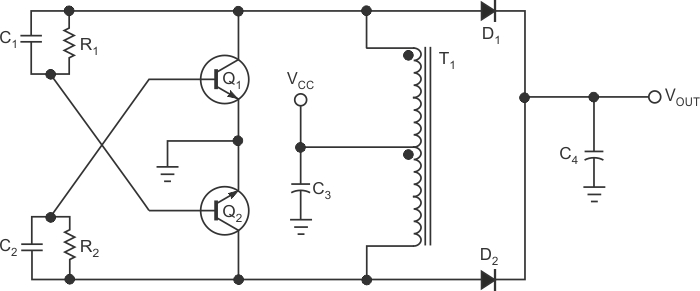Figure 1. A simple one-transformer dc/dc voltage doubler has cross-coupled RC networks from the collectors of Q1 and Q2 to the corresponding bases. These networks provide regenerative feedback.

Figure 1 shows a simple, one-transformer dc/dc converter in which cross-coupled RC networks from the collectors of Q1 and Q2 to the corresponding bases provide regenerative feedback. In operation, the transformer alternates between positive and negative saturation, with collapse in transformer flux leading induced voltages to drive the transistors alternately off and on. The input-voltage and saturation characteristics of the transformer core determine the operating frequency based on the relationship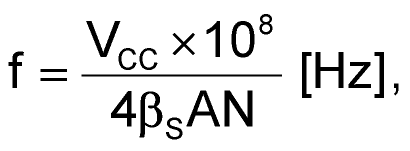where VCC is the input voltage, βS is the saturated flux density in gauss, A is the cross-section area of the core in square centimeters, and N is the number of turns in half of the primary. The circuit uses the property that the collector-to-emitter voltage of each device is approximately twice the supply voltage, VCC, plus induced voltages, which occur because of leakage inductance. Rectification and filtration of the collector voltages of Q1 and Q2 through D1 and D2 directly provide an output voltage that is approximately double the input voltage, VCC.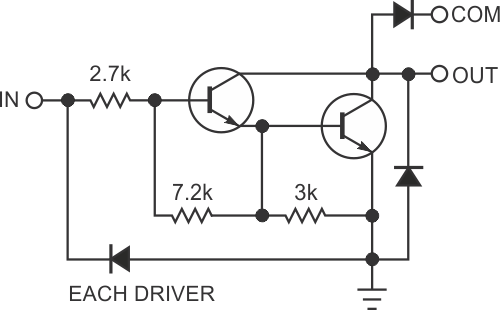Figure 2. The internal configuration of the high-voltage Darlington-array ULN2023A driver exactly matches the requirements of the circuit in Figure 1 by providing rectifier diodes at the collector outputs.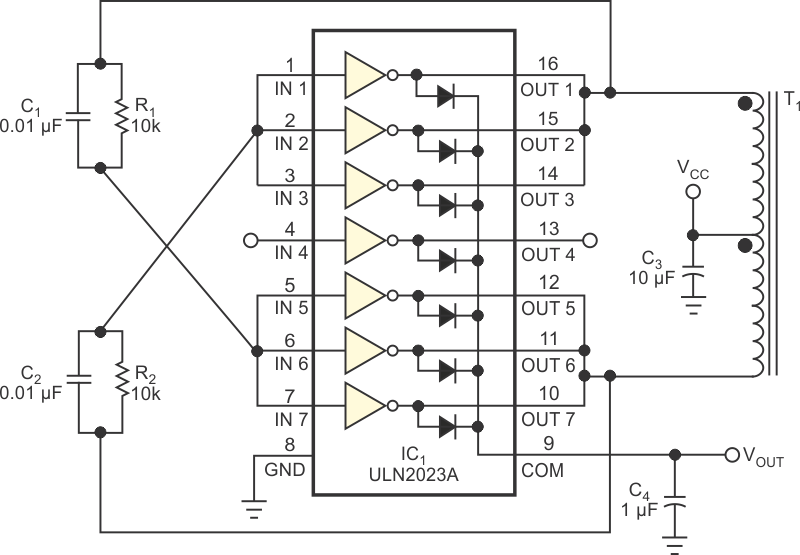Figure 3. Taking advantage of the multiple drivers in one package, three drivers are parallel in each leg of the circuit.

The internal schematic of the high-voltage Darlington-array ULN2023A driver in Figure 2 exactly matches the requirements for the circuit in Figure 1 by providing rectifier diodes at the collector outputs. The voltage-breakdown specification of 95 V meets the maximum requirement of twice VCC plus transients when operating at an input of 30 V. The device exhibits a low collector-to-emitter saturation voltage at the desired current level of approximately 100 mA and low switching times when switching at rates as high as tens of kilohertz.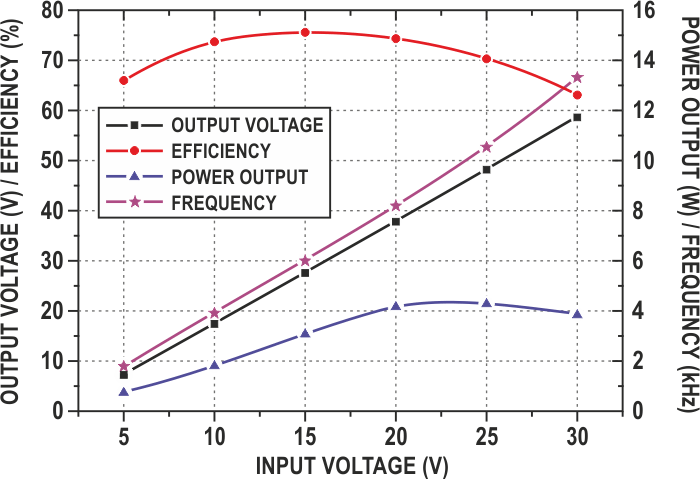Figure 4. Experimental results show the circuit in Figure 3 operating as a low-power, moderately efficient, wide-range voltage doubler.

Figure 3 shows the final circuit configuration. Three drivers operate in parallel, sharing the drive current, minimizing the collector-to-emitter voltage, and maximizing the permitted power dissipation. Table 1 shows the experimental results with the voltage-doubler circuit operating over the input voltage of 5 to 30 V. In that range, the input current is less than 300 mA to remain within the current values of the transformer at lower input voltages and within the power-dissipation limit of the ULN2023A at higher input voltages. Figure 4 shows the plot of the experimental results, clearly indicating the operation of a low-power, moderately efficient, wide-range voltage doubler.

Table 1. Experimental results
 Input voltage (V) Input current (mA) Oscillating frequency (kHz) Output voltage (V) Load current (mA) Power input (W) Power output (W) Efficiency (%) 5 245 1.79 7.59 105.95 1.22 0.8 65.77 10 250 4 17.68 104.13 2.5 1.84 73.72 15 274 6.06 27.7 111.7 4.12 3.09 75.08 20 280 8.2 37.9 110.12 5.6 4.17 74.53 25 242 10.53 48.1 88.23 6.05 4.24 70.15 30 205 13.33 58.7 66.25 6.15 3.89 63.23

Materials on the topic

EDN

You may have to register before you can post comments and get full access to forum.
 User Name Remember Me? Password